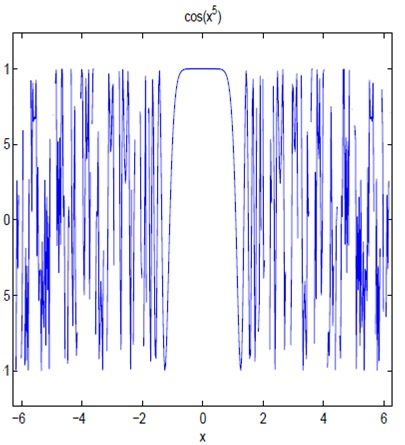#### Symbolic Computations in Matlab

Symbolic Computations:

The focus of this course is on numerical computations that are calculations usually approximations with floating point numbers. Nevertheless Mat lab can as well do symbolic computations which denote exact calculations using symbols as in Algebra or Calculus. You must have done some symbolic Mat lab computations in your Calculus courses and in this chapter we review what you should already know.

Defining functions and basic operations:

Prior to doing any symbolic computation one should declare the variables used to be symbolic:

>syms x y

A function is defined by merely typing the formula:

> f = cos(x) + 3*x^2

Note that coefficients should be multiplied using *. To find specific values you should use the command subs:

>subs(f,pi)

This command denotes for substitute it substitutes π for x in the formula for f.

If we describe another function:

> g = exp(-y^2)

Then we are able to compose the functions:

> h = compose(g,f)

That is h(x) = g(f(x)). Since f as well as g are functions of different variables their product should be a function of two variables:

> k = f*g
>subs(k,[x,y],[0,1])

We can do easy calculus operations like differentiation:

> f1 = diff(f)

Indefinite integrals (ant derivatives):

> F = int(f)

And definite integrals:

>int(f,0,2*pi)

To alter a symbolic answer into a numerical answer utilizes the double command which stands for double precision (not times 2)

> double (ans)

Note that a few ant derivatives can’t be found in terms of elementary functions for some of these it is able to be expressed in terms of special functions:

> G = int(g)

And for others Mat lab does the best it can:Above Figure Graph of cos(x5) produced by the ezplot command. It is incorrect because cos(u) must oscillate smoothly between −1 and 1. The problem amid the plot is that cos(x5) oscillates very rapidly and the plot didn’t consider enough points.

>int(h)

For definite integrals that can’t be evaluated exactly Mat lab does nothing and prints a warning:

>int(h,0,1)

We will observe afterwards those even functions that don’t have an ant derivative can be integrated numerically.

You can alter the last answer to a numerical answer using:

>double(ans)

Plotting a symbolic function is able to be done as follows:

>ezplot(f)

Or the domain can be specified:

>ezplot(g,-10,10)
>ezplot(g,-2,2)

To plan a symbolic function of two variables utilize:

>ezsurf(k)

It is significant to keep in mind that even though we have defined our variables to be symbolic variables plotting can merely plot a finite set of points. For illustration:

>ezplot(cos(x^5))

Will produce a plot that is clearly wrong for the reason that it does not plot enough points.

Other helpful symbolic operations:

Mat lab allows you to do simple algebra. For illustration:

>poly = (x - 3)^5
>polyex = expand(poly)
>polysi = simple(polyex)

To search the symbolic solutions of an equation f(x) = 0 use:

>solve(f)
>solve(g)
>solve(polyex)

Another helpful property of symbolic functions is that you can substitute numerical vectors for the variables:

> X = 2:0.1:4;
> Y = subs(polyex,X);
> plot(X,Y)

Latest technology based Matlab Programming Online Tutoring Assistance

Tutors, at the www.tutorsglobe.com, take pledge to provide full satisfaction and assurance in Matlab Programming help via online tutoring. Students are getting 100% satisfaction by online tutors across the globe. Here you can get homework help for Matlab Programming, project ideas and tutorials. We provide email based Matlab Programming help. You can join us to ask queries 24x7 with live, experienced and qualified online tutors specialized in Matlab Programming. Through Online Tutoring, you would be able to complete your homework or assignments at your home. Tutors at the TutorsGlobe are committed to provide the best quality online tutoring assistance for Matlab Programming Homework help and assignment help services. They use their experience, as they have solved thousands of the Matlab Programming assignments, which may help you to solve your complex issues of Matlab Programming. TutorsGlobe assure for the best quality compliance to your homework. Compromise with quality is not in our dictionary. If we feel that we are not able to provide the homework help as per the deadline or given instruction by the student, we refund the money of the student without any delay.